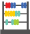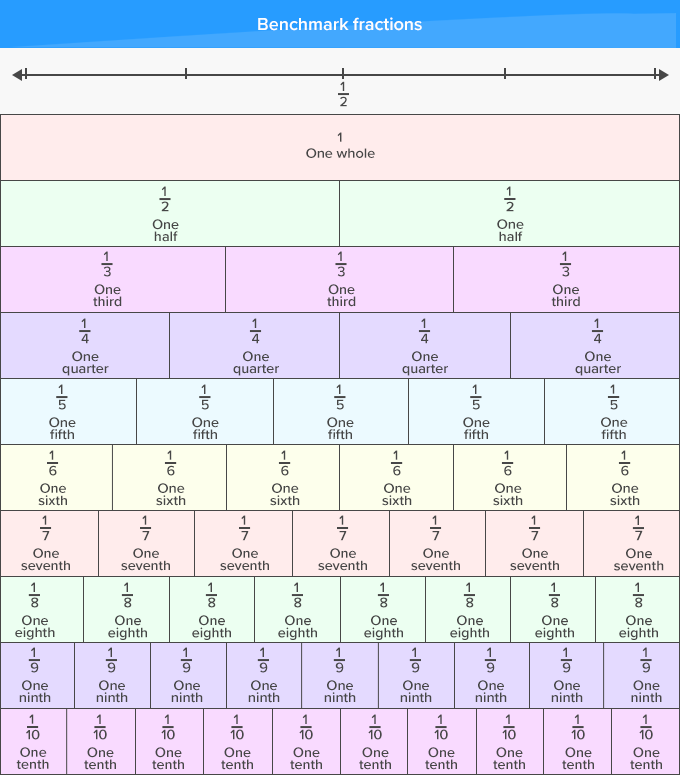# Benchmark Fractions - Definition with Examples

The Complete K-5 Math Learning Program Built for Your Child

• 40 Million Kids

Loved by kids and parent worldwide

• 50,000 Schools

Trusted by teachers across schools

• Comprehensive Curriculum

Aligned to Common Core

##Let's learn!

What is a benchmark fraction?
In math, benchmark fractions can be defined as common fractions that we can measure or judge against, when measuring, comparing or ordering other fractions.

Benchmark fractions are easy to visualize and identify, and thus, help in estimating the parts. When comparing two fractions with different numerators and denominators, we can either make their denominators common or compare them to a benchmark fraction such as 1/2.

Benchmark fractions are most helpful when fractions to be compared are placed on a number line against the benchmarks.

Here’s a chart of benchmark fractions placed on a number line, which can help in comparing fractions.Fun Facts The most commonly used number lines is a ruler. It has halves, fourths, and eighths as benchmarks.

##Let's sing!

When comparing or ordering two different fractions,
Let benchmark fractions be your guiding action.
Just begin by putting the fractions on a number line
Then, choose the benchmark to compare - It’ll be fine!

##Let's do it!

Before you hand out worksheets on comparing fractions, draw the benchmark fractions chart on a chart paper. Give examples and model how the chart can be used to compare and order fractions with different numerators and denominators. You may further give them paper and crayons to draw and represent the fractions to be compared.

##Related math vocabulary

Won Numerous Awards & Honors